Next: Preconditioning for accelerated convergence Up: Interpolation of bathymetry data Previous: Introduction

# Attenuation of the noise bursts and glitches

Now, a formulation of the regridding problem is developed. Let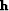be an abstract vector containing as components the water depth over a 2-D spatial mesh and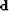be an abstract vector whose successive components represent depth along the vessel tracks. One way to grid irregular data is to minimize the length of the residual vector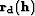(34)
where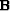is a 2-D linear interpolation operator and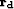is the data residual. Note that sinc or spline interpolants could be used as well, but a simple linear interpolation operator is chosen to focus the analysis on the noise attenuation problem only. This fitting goal simply requires that the gridded datatake on appropriate values where the datawas collected. The bin size is 60 by 50 meters so that the number of data-points per bin is roughly constant and the aspect ratio of the lake is preserved in the number of samples in the east-west and north-south directions. A simple binning (without interpolation or inversion) of the raw data (Figure) is shown in Figure. Note that the north points to the top of the map in Figure. A coarser mesh would avoid the empty bins but lose resolution. As we refine the mesh for more detail, the number of empty bins grows as does the care needed in devising a technique for filling them. The black lines in Figureare the ship tracks. Notice that some data points are outside the contour of the water. These must represent navigation errors.fig1b
Figure 2
Simple binning of the raw data in Figure. The ship tracks and empty bins are visible and need to be accounted for in the inversion process. The north points to the top.Unless data is collected everywhere on a very fine mesh, and depending on how the grid is parameterized, the regridding may leave holes on the mesh. We can eliminate the holes by adding some regularization, like(35)
where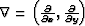and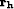is the model space residual. We then minimize the misfit function(36)
to estimate the interpolated map of the lake. The second term in equation () becomes Laplace's equation. In theory Tarantola (1987), the regularization operator (squared) should be the inverse model covariance operator given an a-priori model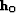. Since we do not have any a-priori model, I simply chose the gradient operator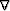as a way of saying that the bottom of the lake is smooth. However, as pointed out by Harlan (1995), the regularization and the data fitting goal in equation () contradict each other. One equation tends to add details in the final map whereas the second one (the regularization) tends to smooth it. We can more easily balance these two goals by preconditioning the problem Fomel (2001).